# Multiple Linear Regression in Excel tutorial

2019-01-30

This tutorial will help you set up and interpret a multiple linear regression in Excel using the XLSTAT software. Linear regression is based on Ordinary Least Squares (OLS).
Not sure this is the modeling feature you are looking for? Check out this guide

## Data to run a multiple linear regression

An Excel sheet with both the data and the results can be downloaded by clicking on the button below:

The data have been obtained in Lewis T. and Taylor L.R. (1967). Introduction to Experimental Ecology, New York: Academic Press, Inc.. They concern 237 children, described by their gender, age in months, height in inches (1 inch = 2.54 cm), and weight in pounds (1 pound = 0.45 kg).

## Goal of this tutorial

Using simple linear regression, we want to find out how the weight of the children varies with their height, and to verify if a linear model makes sense.

The Linear Regression method belongs to a larger family of models called GLM (Generalized Linear Models), as do the ANCOVA and ANOVA. This dataset is also used in the two tutorials on simple linear regression and ANCOVA.

## Setting up a multiple linear regression

After opening XLSTAT, select the XLSTAT / Modeling data / Regression function.Once you've clicked on the button, the Linear Regression dialog box appears.

Select the data on the Excel sheet. The Dependent variable (or variable to model) is here the "Weight".

The quantitative explanatory variables are the "Height" and the "Age".

As we selected the column title for the variables, we leave the option Variable labels activated.In the Outputs tab we activate the Type I/III SS option in order to display the corresponding results.The computations begin once you have clicked on OK. The results will then be displayed.

## Interpreting the results of a multiple linear regression

The first table displays the goodness of fit coefficients of the model. The R² (coefficient of determination) indicates the % of variability of the dependent variable which is explained by the explanatory variables. The closer to 1 the R² is, the better the fit.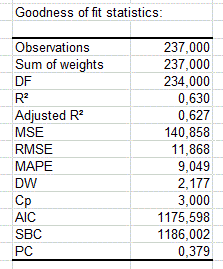In this particular case, 63 % of the variability of the Weight is explained by the Height and the Age. The remainder of the variability is due to some effects (other explanatory variables) that have not been included in this analysis.

It is important to examine the results of the analysis of variance table (see below). The results enable us to determine whether or not the explanatory variables bring significant information (null hypothesis H0) to the model. In other words, it's a way of asking yourself whether it is valid to use the mean to describe the whole population, or whether the information brought by the explanatory variables is of value or not.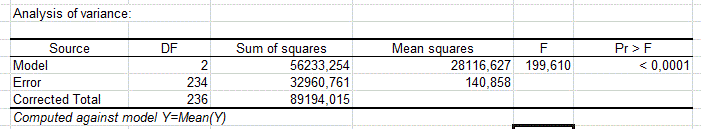The Fisher's F test is used. Given the fact that the probability corresponding to the F value is lower than 0.0001, it means that we would be taking a lower than 0.01% risk in assuming that the null hypothesis (no effect of the two explanatory variable) is wrong. Therefore, we can conclude with confidence that the three variables do bring a significant amount of information.

The next tables display the Type I and Type III SS. These results indicate whether a variable brings significant information or not, once all the other variables are already included in the model.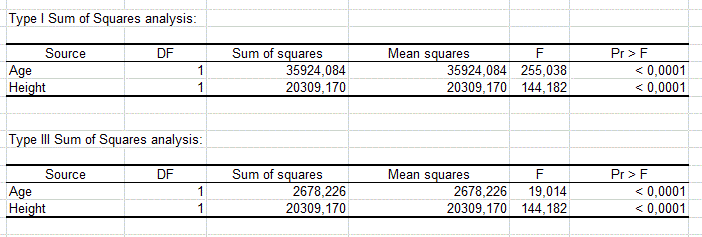The following table gives details on the model. This table is helpful when predictions are needed, or when you need to compare the coefficients of the model for a given population with the ones obtained for another population (it could be used here to compare the models for girls and boys). We can see that the 95 % confidence range of the Height parameter is very narrow, while we notice that the p-value for the Age parameter is much larger than the one of the Height parameter, and that the confidence interval for the Age almost includes 0. This indicates that the Age effect is weaker than the Height effect. The equation of the model is written below the table. We can see that gor a given Height, the age has a positive effect on the Weight: when the Age increases by 1 month, the Weight increases by 0.23 pounds.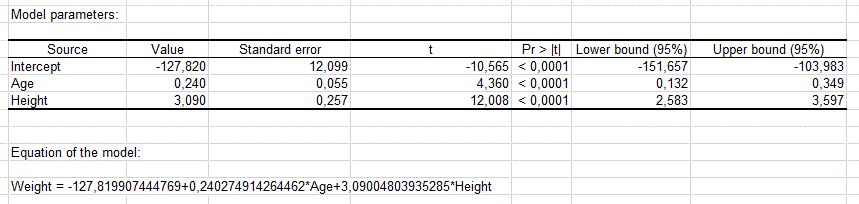The table and the chart below correspond to the standardized regression coefficients (sometimes referred to as beta coefficients). They allow to directly compare the relative influence of the explanatory variables on the dependent variable, and their significance.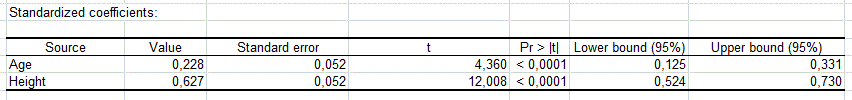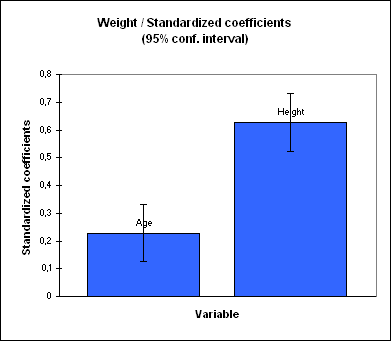The next table shows the residuals. It enables us to take a closer look at each of the standardized residuals. These residuals, given the assumptions of the linear regression model, should be normally distributed, meaning that 95% of the residuals should be in the interval [-1.96, 1.96]. All values outside this interval are potential outliers, or might suggest that the normality assumption is wrong. We used XLSTAT's DataFlagger to bring out the residuals that are not in the [-1.96, 1.96] interval.

Out of 237, we can identify 15 residuals are out of the [-1.96, 1.96] range, which makes 6.3% instead of 5%. A more in depth analysis of the residuals has been performed in a tutorial on ANCOVA The chart below allows us to compare the predicted values to the observed values.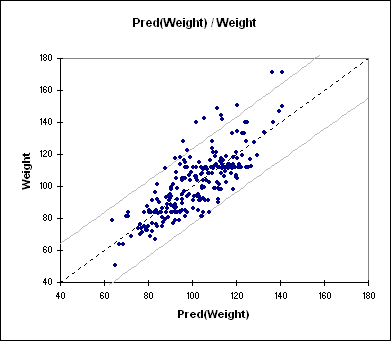The histogram of the residuals enables us to quickly visualize the residuals that are out of the range [-2, 2].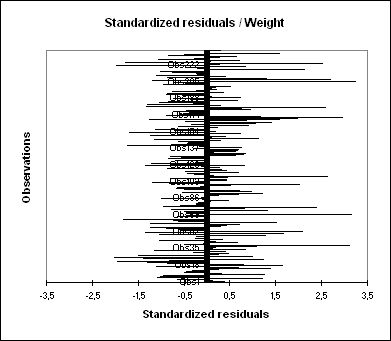## Conclusion for this multiple linear regression

As a conclusion, the Height, the Age and the Gender allow us to explain 63 % of the variability of the Weight. A significant amount of information is not explained by the model we have used. In a tutorial on ANCOVA, the Gender is added to the model to improve the quality of the fit.

The following video explains how to run a multiple linear regression in XLSTAT.

#### Contact our technical support team: support@xlstat.com

https://cdn.desk.com/
false
desk